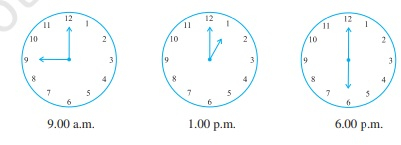# Find the angle measure between the hands of the clock in each figure:"

To do:

We have to find the measure between the hands of the clock in each figure.
Solution:

The measure of the complete angle in a circle is $360^o$.

This implies,

The measure of the angle between any two adjacent numbers $=\frac{360^o}{12}$

$=30^o$

Therefore,

The measure of the angle between the hands of the clock at 9.00 a.m. $=3\times30^o$

$=90^o$

The measure of the angle between the hands of the clock at 1.00 p.m. $=1\times30^o$

$=30^o$

The measure of the angle between the hands of the clock at 6.00 p.m. $=6\times30^o$

$=180^o$